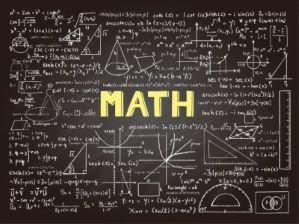# BGCSE MATHEMATICS PART 1\$ 75.00 \$ 50.00Mathematics is the science of numbers, quantities and spaces which can be used for abstract concepts or can be applied to other subjects such as engineering and physics. This course will prepare students to take Paper 1  of the Mathematics Core Level BGCSE. The BGCSE aims to test candidates’ ability to apply and utilize mathematical concepts in a variety of areas such as everyday problem solving and analysis of data. Students will learn basic elements for solving problems. They will also develop an understanding of techniques used to solve these problems in preparation for higher level mathematical examinations.

COURSE OBJECTIVES:

–Develop mathematical knowledge and skills with emphasis on confidence and satisfaction.

–To understand problem solving techniques used to solve equations and word problems.

–Develop a feel for performing operations and demonstrating the importance of results found.

–To recognize how a situation can be represented mathematically and identifying the relevant factors used to arrive at a solution.

–Develop the ability to generalize, prove and classify different aspects of mathematical expressions.

–To produce an appreciation for patterns and the relationships between mathematical concepts.

–To acquire a foundation appropriate for further study of mathematics and its branch disciplines.

### Course Features

• Lectures 17
• Quizzes 0
• Duration 50 hours
• Skill level All levels
• Language English
• Students 0
• Assessments Yes